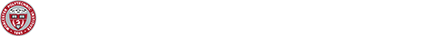# Graphon Mean Field Games: A Dynamical Equilibrium Theory for a Networked World

## Graphon Mean Field Games: A Dynamical Equilibrium Theory for a Networked World

Speaker: Peter Caines from McGill University

Date and Time: 03/06/20, 11am-12

Room: SH203

Abstract: The complexity of large population multi-agent dynamical systems, such as occur in economics, communication systems, and environmental and transportation systems, makes centralized control infeasible and classical game theoretic solutions intractable.

In this talk we first present the Mean Field Game (MFG) theory of large population systems. Going to the infinite population limit, individual agent feedback strategies exist which yield Nash equilibria. These are given by the MFG equations consisting of (i) a Hamilton-Jacobi-Bellman equation generating the Nash values and the best response control actions, and (ii) a McKean-Vlasov-Fokker-Planck–Kolmogorov equation for the probability distribution of the states of the population, otherwise known as the mean field.

Next we shall introduce Graphon Mean Field Game and Control theory. Very large scale networks linking dynamical agents are now ubiquitous, with examples being given by electrical power grids and social media networks. In this setting, the emergence of the graphon theory of infinite networks has enabled the formulation of the Graphon Mean Field Game equations. Just as for MFG theory, it is the simplicity of the infinite population GMFG strategies which permits their application to otherwise intractable problems involving large populations and networks.